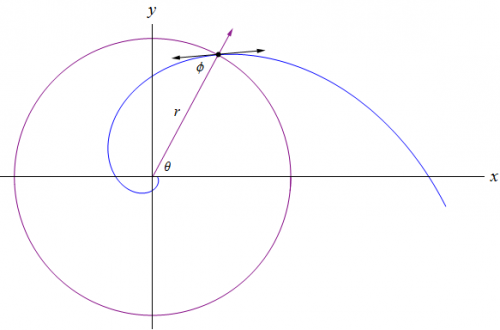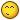## Recommended Posts

I was reminiscing about a problem that my Calculus 3 professor gave us, and decided to modify the problem to make it a little more challenging to solve. Although the problem isn't hard to figure out, it does have some interesting consequences. So without further adieu, I give you my ninth challenge.

A Moth to a Flame

It is common knowledge that certain insects, such as moths, are attracted to light sources. Let's consider that a moth navigates the world by maintaining a constant angle to the sun, which can be considered an infinite light source. Furthermore, let's say that at night the moth uses any light source that is available, and for all intents and purposes our light source is a bug zapper. Since the bug zapper is not an infinite light source, the moth will spiral into the bug zapper due to maintaining a constant angle with respect to radial lines from the center of the bug zapper that define the path of the spiral.The goal of this challenge is to identify the type of spiral, and to find the simplified equation for the curvature of the spiral in terms of the radial distance $d$ and the angle of the moth $\phi$ with respect to radial lines from the center of the bug zapper. Several clues have been given that should help you solve this challenge. Reputation awaits for the one who solves it first.

Edited by Daedalus

##### Share on other sites

"Reputation awaits for the one who solves it first."

How? I don't think he has posted here since he died n years ago

Incidentally n-2 is a square number.

However I guess solving the problem by knowing one of the names of that spiral and googling it doesn't count.

##### Share on other sites

"Reputation awaits for the one who solves it first."

How? I don't think he has posted here since he died n years ago

Incidentally n-2 is a square number.

However I guess solving the problem by knowing one of the names of that spiral and googling it doesn't count.

The problem has two parts. You have to know the type of spiral so you can get it's equation to find the curvature. The equation for it's curvature must be in terms of $d$ and $\phi$. If you know the name of the spiral, go ahead and post it John. Perhaps someone will find the answer by googling it.Edited by Daedalus

##### Share on other sites

Why does your diagram show an r but your wording a d?

Is this intentional misdirection?

Edited by studiot

##### Share on other sites

Why does your diagram show an r but your wording an d?

Is this intentional misdirection?

It's actually a clue that I provided hinting at the way I solved the problem. Nice attention to the details!Edited by Daedalus

##### Share on other sites

It would be more fun to solve the way Descartes originally did.

True, it would be more fun, but the challenge only requires that one provides the equation for the curvature in terms of $d$ and $\phi$.

##### Share on other sites

I'd like to point out that moths do not navigate by the Sun...

##### Share on other sites

I'd like to point out that moths do not navigate by the Sun...

Lol... They sure don't. It's just a set up for the problem as was given to me by my Calc 3 professor. Just to speed things up a little, the spiral is known as a logarithmic or equiangular spiral.

##### Share on other sites

Who actually gave neg rep to Moontanman? Don't worry I'll counter it...

Edited by Daedalus

##### Share on other sites

Who actually gave neg rep to Moontanman? Don't worry I'll counter it...

Because of my willingness of opinion, I did. I didn't see it as a joke rather as a criticism that was unnecessary, therefore I stated that the logic he was presenting was unneeded because it doesn't help solve the problem. The point of a mathematical problem with seemingly illogical parts is to help to solve the problem.

Just my opinion. I will take up the challenge and see if I can solve it.

##### Share on other sites

Well, at least you're honest UnityMoontanman and I are friends, and I took his response as a joke. It's always hard to tell someone's intentions just through text. Feel free to solve the challenge. I found it quite interesting when I worked the problem many years ago, but I love mathematics.

Edited by Daedalus

##### Share on other sites

This curve has some remarkable geometric properties as well as calculus ones. Calculus had not been invented when Descartes discovered it in 1638.

Jacob Bernoulli had one engraved on his tomb along with the words "Eadem metata resurgo"

Because of the constant angle property, if you roll the curve along a straight line, its centre or pole describes another straight line at an angle to the first. Erect a perpendicular from the pole to the first straight line and the length from where it cuts, back along back the first straight line to the starting point is equal to the length of the spiral.

Edited by studiot

##### Share on other sites

This curve has some remarkable geometric properties as well as calculus ones. Calculus had not been invented when Descartes discovered it in 1638.

Jacob Bernoulli had one engraved on his tomb along with the words "Eadem metata resurgo"

Because of the constant angle property, if you roll the curve along a straight line, its centre or pole describes another straight line at an angle to the first. Erect a perpendicular from the pole to the first straight line and the length from where it cuts, back along back the first straight line to the starting point is equal to the length of the spiral.

That's actually pretty cool studiot. The professor who gave us this problem is a good friend of mine. However, he didn't want an equation for the answer. He gave us the distance $d$ and the angle of the moth $\phi$ with respect to radial lines from the center of the bug zapper and wanted the curvature of the logarithmic spiral at that point. So, as usual, I derived the equation and plugged in the numbers. It's actually a pretty neat problem with an interesting result once you try to solve it.

I figured this challenge would be a nice break from my usual type of problems that can be tough to solve. Don't get me wrong. This problem can be challenging too. If you don't know the type of spiral, you would have to search for it online in order to get the equations, which was intended. I didn't expect anyone to go to a library and research the problem to solve it. However, the problem is no where near as tough as my other challenges.Edited by Daedalus

##### Share on other sites

What made you think I went to a Library?

I never thought you went to a library. The comment was intended towards John's statement in the second post:

However I guess solving the problem by knowing one of the names of that spiral and googling it doesn't count.

It's ok to research the problem online, but one would still have to solve for the equation of the curvature in terms of $d$ and $\phi$, which is the whole point of the challenge.

Edited by Daedalus

##### Share on other sites

One of the (not very original) names of that curve is the equiangular spiral.

If you have heard of that and Google it, you get a lot of information about it.

Unfortunately, my maths isn't very good. Can I get some credit for the particular case where= 90 degrees?

##### Share on other sites

Like JC, I didn't want to spell out the name of the curve, until you did, in order not to spoil it for others.

I'm not sure if you seen post #9, but I went ahead and gave everyone the name of the spiral to help speed things up.One of the (not very original) names of that curve is the equiangular spiral.

If you have heard of that and Google it, you get a lot of information about it.

Unfortunately, my maths isn't very good. Can I get some credit for the particular case where= 90 degrees?

Yeah, there is quite a bit of information on the internet concerning logarithmic spirals, but oddly enough I haven't seen the equation for this particular derivation of the curvature. You will find other equations, but they are not in terms using only $d$ and $\phi$. However, that doesn't mean you won't find it, but that it's not listed on the usual sites one would go to look. As for the case when $\phi = 90^\circ$, I included that in the graph I posted as a clue...Edited by Daedalus

##### Share on other sites

Hint the polar form is best.

Most definitely. I ended up converting from polar to parametric to derive the equation for the curvature because I like working with vector valued functions.

Edited by Daedalus

##### Share on other sites

It has been over two weeks and no one has provided the solution. So, here is the answer to this challenge:

A logarithmic spiral is defined by the polar equation:

$r=a\,e^{b\,\theta}$

The following definition as provided by Wikipedia will allow us to solve for $b$ in terms of $\phi$:

$\phi=\text{cos}^{-1}\frac{\left\langle r(\theta),\,r'(\theta)\right\rangle}{\left\|r(\theta)\right \|\,\left\|r'(\theta)\right\|}=\text{tan}^{-1}\frac{1}{b}$

Solving for $b$, we get:

$b=\text{cot}\,\phi$

Now that we have $b$ in terms of $\phi$, we'll substitute the result into the polar equation:

$r=a\,e^{\theta\,\text{cot}\,\phi}$

Now if it isn't obvious what to do with that $a$ to get $d$ into the equation, you can always rewrite the equation using $d$. We'll even add an angle $\varphi$ where the radial distance will be equal to $d$:

$d=a\,e^{\varphi\,\text{cot}\,\phi}$

Solving for $a$, we get:

$a=d\,e^{-\varphi\,\text{cot}\,\phi}$

Substitute the result into the original equation and simplify:

$r=\left(d\,e^{-\varphi\,\text{cot}\,\phi}\right)e^{\theta\,\text{cot}\,\phi}$

$r=d\,e^{(\theta-\varphi)\,\text{cot}\,\phi}$

We can clearly see that when $\theta$ is equal to $\varphi$, the function simplifies to $r=d$. This brings us to the realization that $a$ is just a scaling factor and we didn't need $\varphi$. We could've just defined $r=d$ when $\theta=0$. Next, we'll define the polar function as a vector valued function in order to find the curvature:

$R(\theta)=\left\langle d\,e^{\theta\,\text{cot}\,\phi}\text{cos}\,\theta,\,d\,e^{\theta\,\text{cot}\,\phi}\text{sin}\,\theta\right\rangle$

The curvature of our function $R$ is defined by:

$k(\theta)=\frac{\left\vert T'(\theta)\right\vert}{\left\vert R'(\theta)\right\vert}$

The magnitude of $R'(\theta)$ is equal to:

$\left\vert R'(\theta)\right\vert=d\,e^{\theta\,\text{cot}\,\phi}\text{csc}\,\phi$

Solving for the unit tangent vector $T$, we get:

$T(\theta)=\frac{R'(\theta)}{\left\vert R'(\theta)\right\vert}=\left\langle\text{cos}(\theta+\phi),\,\text{sin}(\theta+\phi)\right\rangle$

The magnitude of $T'(\theta)$ is equal to one:

$\left\vert T'(\theta)\right\vert=\sqrt{\left(-\text{sin}(\theta+\phi)\right)^2+\left(\text{cos}(\theta+\phi)\right)^2}=1$

Now that we have $\left\vert R'(\theta)\right\vert$ and $\left\vert T'(\theta)\right\vert$, we can define the curvature:

$k(\theta)=\frac{1}{d\,e^{\theta\,\text{cot}\,\phi}\text{csc}\,\phi}$

As stated, when $\theta=0$ the function simplifies to $r=d$. So, we can set $\theta=0$ and arrive at the solution for the challenge:

$k=\frac{1}{d\,\text{csc}\,\phi}=\frac{\text{sin}\,\phi}{d}$

A circle also has this equiangular property and its curvature is $k=1/d$, which is what we expect for $\phi=\pi/2$ or $90^\circ$:

$k=\frac{\text{sin}(\pi/2)}{d}=\frac{1}{d}$

Ninth Challenge.zip

Edited by Daedalus

## Create an account

Register a new account